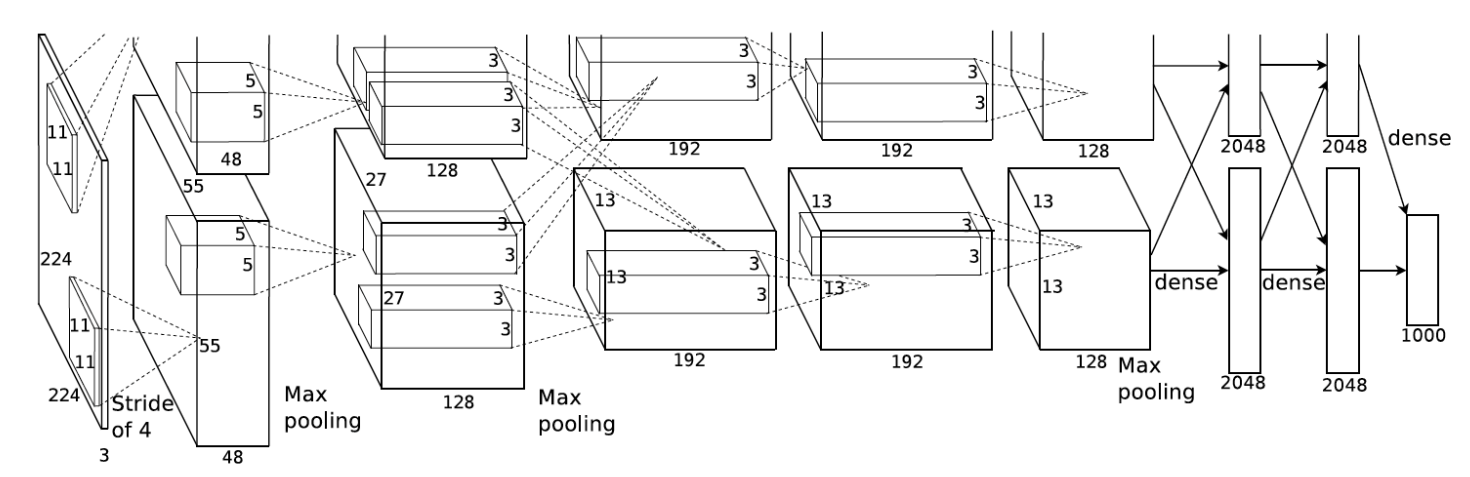# PyTorch实战福利从入门到精通之八——深度卷积神经网络（AlexNet）

1. 获取图像数据集；
2. 使用已有的特征提取函数生成图像的特征；
3. 使用机器学习模型对图像的特征分类。

2012年，AlexNet横空出世。这个模型的名字来源于论文第一作者的姓名Alex Krizhevsky 。AlexNet使用了8层卷积神经网络，并以很大的优势赢得了ImageNet 2012图像识别挑战赛。它首次证明了学习到的特征可以超越手工设计的特征，从而一举打破计算机视觉研究的前状。模型的选择对图像分类结果的影响更大。AlexNet与LeNet的设计理念非常相似，但也有显著的区别。

AlexNet第一层中的卷积窗口形状是$11\times11$。因为ImageNet中绝大多数图像的高和宽均比MNIST图像的高和宽大10倍以上，ImageNet图像的物体占用更多的像素，所以需要更大的卷积窗口来捕获物体。第二层中的卷积窗口形状减小到$5\times5$，之后全采用$3\times3$。此外，第一、第二和第五个卷积层之后都使用了窗口形状为$3\times3$、步幅为2的最大池化层。而且，AlexNet使用的卷积通道数也大于LeNet中的卷积通道数数十倍。

import time
import torch
from torch import nn, optim
import torchvision

import sys
sys.path.append("..")
import d2lzh_pytorch as d2l
device = torch.device('cuda' if torch.cuda.is_available() else 'cpu')

class AlexNet(nn.Module):
def __init__(self):
super(AlexNet, self).__init__()
self.conv = nn.Sequential(
nn.Conv2d(1, 96, 11, 4), # in_channels, out_channels, kernel_size, stride, padding
nn.ReLU(),
nn.MaxPool2d(3, 2), # kernel_size, stride
# 减小卷积窗口，使用填充为2来使得输入与输出的高和宽一致，且增大输出通道数
nn.Conv2d(96, 256, 5, 1, 2),
nn.ReLU(),
nn.MaxPool2d(3, 2),
# 连续3个卷积层，且使用更小的卷积窗口。除了最后的卷积层外，进一步增大了输出通道数。
# 前两个卷积层后不使用池化层来减小输入的高和宽
nn.Conv2d(256, 384, 3, 1, 1),
nn.ReLU(),
nn.Conv2d(384, 384, 3, 1, 1),
nn.ReLU(),
nn.Conv2d(384, 256, 3, 1, 1),
nn.ReLU(),
nn.MaxPool2d(3, 2)
)
# 这里全连接层的输出个数比LeNet中的大数倍。使用丢弃层来缓解过拟合
self.fc = nn.Sequential(
nn.Linear(256*5*5, 4096),
nn.ReLU(),
nn.Dropout(0.5),
nn.Linear(4096, 4096),
nn.ReLU(),
nn.Dropout(0.5),
# 输出层。由于这里使用Fashion-MNIST，所以用类别数为10，而非论文中的1000
nn.Linear(4096, 10),
)

def forward(self, img):
feature = self.conv(img)
output = self.fc(feature.view(img.shape, -1))
return output

AlexNet(
(conv): Sequential(
(0): Conv2d(1, 96, kernel_size=(11, 11), stride=(4, 4))
(1): ReLU()
(2): MaxPool2d(kernel_size=3, stride=2, padding=0, dilation=1, ceil_mode=False)
(3): Conv2d(96, 256, kernel_size=(5, 5), stride=(1, 1), padding=(2, 2))
(4): ReLU()
(5): MaxPool2d(kernel_size=3, stride=2, padding=0, dilation=1, ceil_mode=False)
(6): Conv2d(256, 384, kernel_size=(3, 3), stride=(1, 1), padding=(1, 1))
(7): ReLU()
(8): Conv2d(384, 384, kernel_size=(3, 3), stride=(1, 1), padding=(1, 1))
(9): ReLU()
(10): Conv2d(384, 256, kernel_size=(3, 3), stride=(1, 1), padding=(1, 1))
(11): ReLU()
(12): MaxPool2d(kernel_size=3, stride=2, padding=0, dilation=1, ceil_mode=False)
)
(fc): Sequential(
(0): Linear(in_features=6400, out_features=4096, bias=True)
(1): ReLU()
(2): Dropout(p=0.5)
(3): Linear(in_features=4096, out_features=4096, bias=True)
(4): ReLU()
(5): Dropout(p=0.5)
(6): Linear(in_features=4096, out_features=10, bias=True)
)
)

虽然论文中AlexNet使用ImageNet数据集，但因为ImageNet数据集训练时间较长，我们仍用前面的Fashion-MNIST数据集来演示AlexNet。读取数据的时候我们额外做了一步将图像高和宽扩大到AlexNet使用的图像高和宽224。这个可以通过torchvision.transforms.Resize实例来实现。也就是说，我们在ToTensor实例前使用Resize实例，然后使用Compose实例来将这两个变换串联以方便调用。

def load_data_fashion_mnist(batch_size, resize=None, root='~/Datasets/FashionMNIST'):
trans = []
if resize:
trans.append(torchvision.transforms.Resize(size=resize))
trans.append(torchvision.transforms.ToTensor())

transform = torchvision.transforms.Compose(trans)

train_iter = torch.utils.data.DataLoader(mnist_train, batch_size=batch_size, shuffle=True, num_workers=4)
test_iter = torch.utils.data.DataLoader(mnist_test, batch_size=batch_size, shuffle=False, num_workers=4)

return train_iter, test_iter

batch_size = 128
# 如出现“out of memory”的报错信息，可减小batch_size或resize
train_iter, test_iter = load_data_fashion_mnist(batch_size, resize=224)

lr, num_epochs = 0.001, 5
d2l.train_ch5(net, train_iter, test_iter, batch_size, optimizer, device, num_epochs)

training on  cuda
epoch 1, loss 0.0047, train acc 0.770, test acc 0.865, time 128.3 sec
epoch 2, loss 0.0025, train acc 0.879, test acc 0.889, time 128.8 sec
epoch 3, loss 0.0022, train acc 0.898, test acc 0.901, time 130.4 sec
epoch 4, loss 0.0019, train acc 0.908, test acc 0.900, time 131.4 sec
epoch 5, loss 0.0018, train acc 0.913, test acc 0.902, time 129.9 sec

## 小结

• AlexNet跟LeNet结构类似，但使用了更多的卷积层和更大的参数空间来拟合大规模数据集ImageNet。它是浅层神经网络和深度神经网络的分界线。
• 虽然看上去AlexNet的实现比LeNet的实现也就多了几行代码而已，但这个观念上的转变和真正优秀实验结果的产生令学术界付出了很多年。

02-179902-21211
08-18339
01-0747
03-19190
11-144万+
07-253万+
05-292079
09-28610
11-201211
02-181210
02-18476
01-052万+
06-2162
08-141498
05-09147
01-209万+
01-29358